# Difference between revisions of "7"

## Summary

### Factorization

The number 7 is a prime number.

### Properties and families

Property or family Parameter values First few numbers Proof of satisfaction/membership/containment
prime number fourth prime number 2, 3, 5, 7, 11, 13, 17, 19, 23, 29, 31, 37, 41, 43, 47, 53, 59, 61, 67, 71, 73, 79, 83, 89, 97, [SHOW MORE]View list on OEIS divide and check
Mersenne number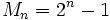, i.e.,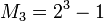plug and check
Mersenne prime (both a prime number and a Mersenne number) same as for Mersenne number combine above
safe prime (odd prime such that half of that minus one is also prime) second safe prime 5, 7, 11, 23, 47, 59, 83, 107, 167, [SHOW MORE]View list on OEIS plug and check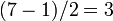is prime.
regular prime third regular prime 3, 5, 7, 11, 13, 17, 19, 23, 29, 31, 41, 43, 47, 53, 61, [SHOW MORE]View list on OEIS

## Structure of integers mod 7

### Discrete log

3 is a primitive root mod 7, so we can take that as the base of the discrete logarithm. We thus get an explicit bijection from the additive group of integers mod 6 to the multiplicative group of nonzero congruence classes mod 7, given by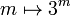. The inverse of that mapping is the discrete logarithm, i.e., the discrete logarithm ofismeans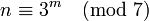:

Congruence class mod 7 (written as smallest positive integer) Congruence class mod 7 (written as smallest magnitude integer) Discrete logarithm to base 3, written as integer mod 6 Is it a primitive root mod 7 (if and only if the discrete log is relatively prime to 6)? Is it s quadratic residue or nonresidue mod 7 (residue if discrete log is even, nonresidue if odd)
1 1 0 No quadratic residue
2 2 2 No quadratic residue
3 3 1 Yes quadratic nonresidue
4 -3 4 No quadratic residue
5 -2 5 Yes quadratic nonresidue
6 -1 3 No quadratic nonresidue

Alternatively, we could take discrete logs to base 5, which is the other primitive root. This is simply the negative of the other discrete log:

Congruence class mod 7 (written as smallest positive integer) Congruence class mod 7 (written as smallest magnitude integer) Discrete logarithm to base 5, written as integer mod 6 Is it a primitive root mod 7 (if and only if the discrete log is relatively prime to 6)? Is it s quadratic residue or nonresidue mod 7 (residue if discrete log is even, nonresidue if odd)
1 1 0 No quadratic residue
2 2 4 No quadratic residue
3 3 5 Yes quadratic nonresidue
4 -3 2 No quadratic residue
5 -2 1 Yes quadratic nonresidue
6 -1 3 No quadratic nonresidue